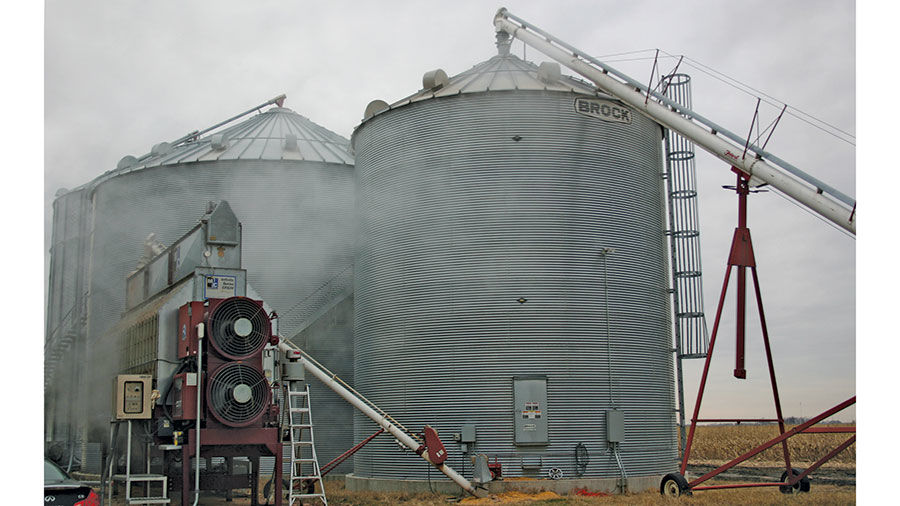By far the largest cost components for drying grain will be fuel and/or electricity, so these costs should receive the most attention when estimating total costs. File photo

Editor’s note: The following was written by William Edwards, Iowa State University retired economist, for the university’s Ag Decision Maker website.

The cost of drying corn grain will depend on the type and size of drying system utilized, the amount of moisture to be removed, the weather conditions during the drying period, and the current per unit costs for electricity and drying fuel.

Depreciation and interest

The initial investment cost of a drying system includes the purchase price of the drying unit itself plus any needed augers, wiring, concrete and other amenities. Their total cost should be amortized over the expected life of the system.

Converting the up-front investment into a series of payments provides an estimate of the annual cost of the system. It is equivalent to repaying a 100% loan over the life of the system, or placing funds into a savings account each year to replace the system at the end of its life. This annual “payment” includes both depreciation and interest costs in a single value.

A useful life of 20 to 25 years is reasonable for a grain drying system, although many systems provide service for longer periods. The interest rate used can be the current rate charged on long-term farm equipment loans or an average of the borrowing rate and the rate that could be earned on the farm’s equity capital.

Repair and maintenance

The cost of performing annual maintenance and fixing or replacing components in a drying system is usually relatively low. Extension specialists suggest using 3% of the initial investment cost as an estimate of annual repair and maintenance costs. However, this will vary with the amount of use the system has each year.

Fuel cost

The amount of fuel used to dry grain will vary widely with the type of system employed. Extension specialists suggest an average consumption of 0.018 gallons of liquid propane (LP) fuel for each percentage point of moisture removed from a bushel of corn for high-temperature drying systems, with a range of 0.010 to 0.025 gallons.

The assumed usage rate is multiplied by the number of points of moisture to be removed from each bushel, then by the number of bushels to be dried, then finally by the price per gallon of LP gas.

Electricity Cost

Most drying systems also use electricity to power fans, move grain and in some cases provide heat. Estimate an average electricity usage of 0.01 kilowatt-hour (kWh) per point of moisture removed per bushel for high-temperature systems.

For natural air drying systems, which use only electricity, the estimate is an average usage rate of 0.33 kWh per point removed, with a range of 0.30 to 0.40 kWh. The assumed usage rate is multiplied by the number of points of moisture to be removed from each bushel, then by the number of bushels to be dried, then finally by the price per kWh of electricity.

Handling and labor

Moving grain into and out of the drying system generally requires some power cost, whether electrical or by mechanical means, though the cost is usually small.

The amount of labor needed to operate a drying system will vary considerably with the type of system. A high-temperature, fast-drying system may require frequent monitoring, while a slower system may need to be checked much less often.

The labor cost per bushel dried can be estimated by dividing a typical farm labor wage rate per hour by the number of bushels typically dried in an hour by the system, then multiplying by the percent of the total drying time that labor is needed.

Total cost

The total of annual depreciation and interest, plus maintenance and repair costs can be divided by the number of bushels dried annually to estimate a fixed cost per bushel. This value can be added to the cost per bushel for labor and handling.

Dividing this total by the number of points of moisture typically removed provides an estimate of cost per point. This can be added to the cost per point for fuel and electricity to find a total cost per point of moisture removed.

This value can be used to compare among different drying systems, or to a commercial drying charge. By far the largest cost components for drying grain will be fuel and/or electricity, so these costs should receive the most attention when estimating total costs.